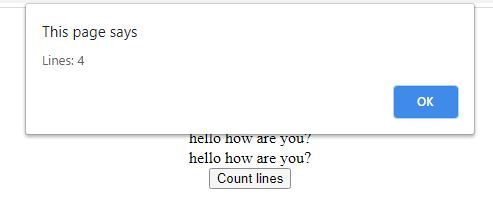Related Articles
How to count text lines inside of DOM element ?
• Last Updated : 12 Jun, 2020

To count number of text lines inside DOM element, we will use the following approach.

1. Obtain the total height of content inside the DOM element.
2. Obtain the height of one line.
3. By dividing the total height of the content by the height of one line, you get the total number of lines inside the element.

Example:

## html

 ```<``html``>`` ` `<``head``>``    ``<``title``>Count lines ```` ` `<``body``>``    ``<``h1` `style``=``"color: green;"``>``        ``GeeksForGeeks``    ```` ` `    ``<``div` `id``=``"content"` `style="width: 100%; ``                ``line-height: 20px">``        ``hello how are you?<``br``>``        ``hello how are you?<``br``>``        ``hello how are you? <``br``>``        ``hello how are you?<``br``>``    ```` ` `    ``<``button` `onclick``=``"countLines()"``>``        ``Count lines``    ```` ` `    ``<``script``>`` ` `        ``// Function to count total``        ``// number of lines``        ``function countLines() {`` ` `            ``// Get element with 'content' as id                            ``            ``var el = ``                ``document.getElementById('content');`` ` `            ``// Get total height of the content    ``            ``var divHeight = el.offsetHeight`` ` `            ``// object.style.lineHeight, returns ``            ``// the lineHeight property``            ``// height of one line ``            ``var lineHeight = ``                ``parseInt(el.style.lineHeight);`` ` `            ``var lines = divHeight / lineHeight;``            ``alert("Lines: " + lines);``        ``}``    `````` ` ``
• Before Clicking on the button:• After Clicking on the button:My Personal Notes arrow_drop_up